Today, we'll be taking a look at the function of a calculator button that may remain a mystery to those but a bold few who choose to study mathematics and calculus: The "log" button!

This is going to be another algebra heavy topic, so if you're not already comfortable working with algebra, perhaps it's best to brush up on that first, and then come back.

So, what is this button? "log" is short for "logarithms", which we use - in combination with exponents - to express large numbers and calculations. However, there's a catch: there is a set of laws surrounding logarithms and exponents, known as the "laws of logarithms"; if we don't follow these laws, then things will go wrong when we try to solve any equations.

Throughout the duration of your course, you'll find logarithms most useful when solving exponential equations, and you'll see why below!

Without further ado, let's get underway looking at some of the logarithm laws, and how you might use them in practice to solve tricky questions.The best Maths tutors available4.9 (59 reviews)
Richa
₹900
/h1st class free!5 (61 reviews)
Rahul
₹1,000
/h1st class free!5 (32 reviews)
Jasleen
₹800
/h1st class free!4.9 (16 reviews)
Faizan
₹500
/h1st class free!5 (52 reviews)
Ranjan
₹500
/h1st class free!5 (20 reviews)
Khushi
₹600
/h1st class free!4.9 (14 reviews)
Preeti
₹2,000
/h1st class free!4.9 (18 reviews)
Shilpa
₹600
/h1st class free!4.9 (59 reviews)
Richa
₹900
/h1st class free!5 (61 reviews)
Rahul
₹1,000
/h1st class free!5 (32 reviews)
Jasleen
₹800
/h1st class free!4.9 (16 reviews)
Faizan
₹500
/h1st class free!5 (52 reviews)
Ranjan
₹500
/h1st class free!5 (20 reviews)
Khushi
₹600
/h1st class free!4.9 (14 reviews)
Preeti
₹2,000
/h1st class free!4.9 (18 reviews)
Shilpa
₹600
/h1st class free!

## The Exponential Function

Let's get a special case out of the way first. You've probably come across the exponential function already, it's simply written using notation like "ex", and you may see it in an expression like so:

In case you aren't too familiar with exponents, they hold a special property that makes them useful... The derivative of  "e" (which is short for "exponential form") is equal to itself, that is to say, if we were to differentiate (learn more about Differentiation/integration problems here) the expression above, we'd end up with the following:

When we use logarithms (also known as logarithmic functions), we typically use the "ln" notation to represent what's known as the "natural log" - this is the inverse function of the exponent. What exactly is the exponent? It's what's known as a "constant" because it never changes. "e" represents a specific real number with a lot of decimal places, so it's easier to just represent it using a common notation like a single letter. Just like we do for Pi!

Before we get started looking at the meaty part of logarithm rules, let's do a really quick sample question using natural logs.

### Natural Logarithm Example

Here's an example question:

For the exponential equation below, solve for x: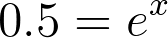This looks tricky on the face of it, but only because we have an "e" (exponential) thrown into the mix. I think we can all agree that once that's out of the picture, everything will get a lot easier. But how to go about that?

Well, thankfully "ln" has another handy property: it's the inverse of "e", this means that by adding it into our equation, we can cancel out the exponential - just like you might divide an equation by a number to get rid of a coefficient.

Let's do that now: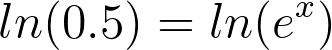Remember, with equations everything we do on one side of the equation, has to be done on the other. Now, "ln" and "e" are going to cancel each other out, just like multiplying and then dividing by the same number would.

Once that's out of the way, we'll have our answer: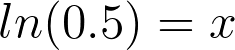Our exponential equation has now been solved! We don't need to do anything else with the ln(...), as this represents a real number - put it into any graphing or scientific calculator and see!

Note: Get a reputable math online tutoring on Superprof.

## The Laws of Logarithms

Down to business: let's get into the nitty-gritty of logs!

There's something to bear in mind when looking at logarithm laws - in all the examples below, we just use "loga", the notation normally used with logarithms is either "log", when the logarithm function is using base10, or "loga", where "a" is a number, when the logarithm is using a different base.

Any law can be applied to any base system, provided there's no change of base in the expression. For example, all these laws apply to base3 numbers as well, as long as you use base3 for every number in your expression.

### Log Law No1

The first law states that adding the logarithms of two numbers (of the same base!) together is the same as taking the logarithm after we multiply the numbers together.

### Log Law No2

The second law states that subtracting the logarithms of two numbers (again, of the same base), is equivalent to dividing the two numbers and taking the logarithm of the result.

### Log Law No3

The final logarithm law we'll look at may seem familiar to you - it's very similar to differentiating a term of an expression! The logarithm of a number raised to a power is the same as the whole logarithm being multiplied by that exponent, removing the exponent from the original expression.

## Putting It All Together

These all look pretty simple in isolation, but unfortunately, it's rarely so easy in an exam. Normally you'll be faced with the prospect of using any number of combinations of logarithmic functions in order to come up with an answer.

Questions about the properties of logarithms quite often crop up in the format of simplifying an expression. That is - you'll be given an expression that includes several different logarithmic terms, and you will have to reduce this down as much as possible - normally to just one or two terms.

While this may seem daunting at first, as always, the best way to approach these problems is to tackle them in bitesize chunks: splitting the question into smaller problems will make it seem much more manageable, and simplifying some of the terms may also make completing a trickier part of the question more obvious to you!

With this in mind, let's take a look at an example of simplifying a logarithmic expression. Notice how because this is an expression and not an equation, there's no equals sign - this means that we can't really "solve" expressions, as they're not problems, just a mathematical statement of fact.

### The Example

Here, all of the terms in our expression are in base10, so we don't specify a base. The question is, how can we simplify this? If you're able to spot the first step, then everything else pretty much works itself out.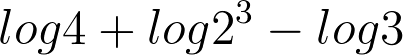All we've done here is rewrite the second logarithmic function, using the third one of the properties of logarithms that we looked at above. This lets us turn the second term of the expression into a polynomial. The second term we've ended up with (and the second term in the original question both evaluate the same) and are just expressed in different ways. If you don't believe me, you can check on a calculator by entering just the second term of the expression.

Do this once for the term we just created, and once for the original term, and you should see they're both the same value.

Now, at the end of the day, all numbers are numbers: this means that we can take our two to the power of three, and turn it onto 8. Let's do that: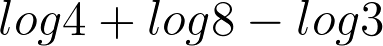This is starting to look nicer. We're getting closer to just being able to perform simple arithmetic. If you're not sure what's next, take a glance up at the logarithm laws we outlined earlier, and see if you can work out where to go from here.

The next step is to simplify even further, by removing another term. We can now use the first logarithm law we learnt, that adding two logarithms together is the same as multiplying their numerical terms. Once we've done this, we'll be left with two logarithms. We're nearly there!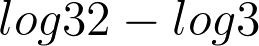We've multiplied the first two numbers together to make 32, and simplify our expression into two logarithms.

Take a look above, you'll see there's only one law left that we haven't used, and hopefully you'll also see that it fits the final stage of our problem perfectly! We can now subtract the two logarithms: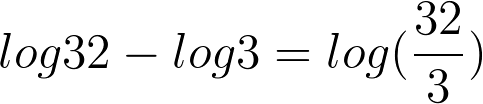We can't simplify that fraction anymore, so our final answer becomes: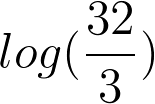And we're done!

## Wrapping Up the Rules of Logs

The good news is that the laws of logarithms are fairly simple in their own right - all you really need is a sound understanding of basic arithmetic and you're well on your way. Where it gets trickier is combining these laws in order to solve more complex problems.

If you find yourself struggling with any of the laws of logarithms, focus on (or make up!) some questions that only require you to use the law you're struggling with, and keep plugging away at it until you feel more comfortable using that particular law in questions.

Logarithms are likely to come up frequently when you're faced with calculus questions, so make sure you're comfortable using them.

As always in math, practice makes perfect! Although these mathematical concepts don't seem like much on their own, they all add up to be essential cogs in a large machine! If you fancy some extra activities involving logarithmic equations and exponent functions, try graphing e(x) and ln(x), and see if you can spot any patterns.

If you do find yourself struggling, you might want to consider using SuperProf to find maths tutors who can give you a helping hand remembering these identities. Don't worry if you're unsure of something, lots of students struggle with all sorts of mathematics problems: from trigonometry to solving quadratic equations!

As well as logarithms, you can find maths help on Calculus and Mechanic Forces for your maths GCSE and A level here.

Check for Vedic Maths tutorial here on Superprof.The platform that connects tutors and students5.00 (1 rating(s))Loading...